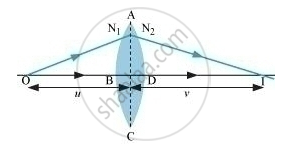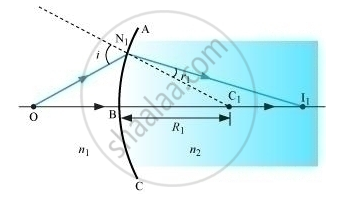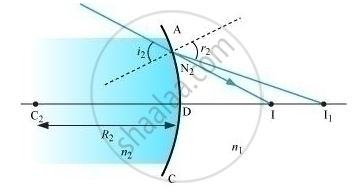Advertisement Remove all ads

# Trace the Rays of Light Showing the Formation of an Image Due to a Point Object Placed on the Axis of a Spherical Surface Separating the Two Media of Refractive Indices N1 and N2.Hence Derive the - Physics

Advertisement Remove all ads
Advertisement Remove all ads
Advertisement Remove all ads

Trace the rays of light showing the formation of an image due to a point object placed on the axis of a spherical surface separating the two media of refractive indices n1 and n2. Establish the relation between the distances of the object, the image and the radius of curvature from the central point of the spherical surface.

Hence derive the expression of the lens maker’s formula.

Advertisement Remove all ads

#### SolutionIn the given figure, image is I and object is denoted as O.

The centre of curvature is C.

The rays are incident from a medium of refractive index n_1to another of refractive indexn_2.

We consider NM to be perpendicular to the principal axis.

tan angleNOM = (MN)/(OM)

tan angleNCM = (MN)/(MC)

tan angleNIM = (MN)/(MI)

For ΔNOC, i is the exterior angle.

Therefore, i = ∠NOM + ∠NCM

i =(MN)/(OM) + (MN)/(MC)

Similarly,

r = ∠NCM − ∠NIM

i.e., r = (MN)/(MC) - (MN)/(MI)

According to Snell’s law,

n_1 sin i = n_2 sin r

For small angles,

n_1i = n_2r

Substituting i and r, we obtain

n_1/(OM) + (n_2)/(MI) = (n_2 - n_1)/(MC)

Where, OM, MI, and MC are the distances

OM = −u

MC = +R

MI = v

Substituting these, we obtain

n_2/v -n_1/u = (n_2 -n_1)/R     ..  (1)

Applying equation (i) to lens ABCD, we obtain for surface ABC,

n_1 /(OB) +n_2/(BI_1) = (n_2 -n_1)/(BC_1)    ... (2)

For surface ADC, we obtain
(-n_2)/(DI_1) + (n_1)/(DI) = (n_2n_1)/(DC_2) ..... (3)

For a thin lens,

BI1 = DI1­

Adding (ii) and (iii), we obtain

n_1/(OB) + n_1/(DI) = (n_2-n_1) [1/(BC_1) +1/(DC_2)]

Suppose object is at infinity and DI = f, then

n_1/f = (n_2 - n_1) [1/(BC_1)+1/(DC_2)]

This is known as lens maker’s formula.

Concept: Thin Lens Formula
Is there an error in this question or solution?

#### APPEARS IN

Advertisement Remove all ads
Share
Notifications

View all notifications

Forgot password?